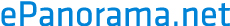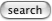# Measuring speaker element parameters

It's possible to get most of the Thiele-Small parameters from a loudspeaker by just accurately measuring the impedance versus frequency. If this is done twice, one with the driver in open air and one with an added (known) mass it should be fairly easy to calculate the fs, Vas and Qt.

What you need is a sine wave generator with reasonably low distortion (<1%), as flat frequency response as possible, and good, stable frequency. You'll need an AC voltmeter that is also flat over the indented range and has the needed sensitivity. A frequency counter is also useful, since the frequency calibration of most oscillators is pretty awful. You'll need an accurate means of measuring DC resistance as well. Add to that a precision 8-10 ohm resistor for calibration purposes, and a 1 kOhm resistor to turn your frequency generator into a virtual current source.

Here's how to proceed:

• Using either an 8 ohm precision resistor (or accurately measuring the resistance of the "calibration" resistor), turn your generator/AC voltmeter into an impedometer by driving the calibrated resistor from the generator through the 1 kOhm resistor, and adjust the output of the generator until you get a convenient voltage across the resistor. For example, if the calibration resistor is 8 ohms, you might adjust the output so that you measure 8 mV across it. Basically you make the current 1 mA so that on the mV scale on your voltmeter the reading is effectively both mV and Ohms. Your setup will look like this:
```                  o---- 1kOhm -----+<-------+
|                |        |
Sine gen.       8 ohms   AC voltmeter
|                |        |
o----------------+<-------+
```
This circuit is called an impedometer, where the voltage across the load is proportional to the impedance of the load. The 1 kohm resistor turns the oscillator into a pretty good approximation of constant-current source.
• Measure the DC resistance of the driver to test. This gives you Re. [Let's say it's 6.5 ohms]
• Replace the calibration resistor with the driver to test. Do not change the voltage from the generator!
• Adjust the frequency in the region of the specified resonance until the voltage across the driver is at a MAXIMUM. Record the frequency. This is Fs, the resonant frequency [let's say it's 32 Hz]. Also, measure the voltage across the driver. This is defined by Re+Res. [let's say voltage is 42 mV which means Re+Res is 42 ohms]. (using an oscilloscope set for phase measurement, Fs will also be where the phase is 0).
• Calculate the ratio between the DC resistance (Re) and the maximum impedance (Re+Res), call it Rc. [In this case, it will be 42/6.5 or 6.46]
• Find the two frequencies on either side of the resonant frequency f1 and f2 where the impedance is Re * sqrt(Rc) [in this example, that impedance will be 6.5 * sqrt(6.46) = 16.5 ohms, and let's say that occurs at f1 = 22.6 Hz and f2 = 45.3 Hz].
• Calculate Qms as:
```
Fs sqrt(Rc)
Qms = -----------
f2 - f1

[in the example, it will be:

32 sqrt(6.46)      32 * 2.54      81.3
Qms = -------------  =  -----------  =  ----  =  3.58
45.3 - 22.6          22.7        22.7

```
• Calculate Qes as:
```
Qms
Qes = --------
(Rc - 1)

[in this example, it will be:

3.58         3.58
Qes = ---------  =  ------  =  0.66
6.46 - 1       5.46

```
• Calculate Qts as:
```
Qes * Qms
Qts = ---------
Qes + Qms

[here, it would be:

0.66 * 3.58      2.36
Qts = -----------  =  ------  =  0.56
0.66 + 3.58      4.24

```
So, you have derived Fs, Res, Qms, Qes, Qts for the driver.
• Repeat the measurements in a sealed, leak-free, unlined test box, and determine the equivalent values of Fc, Qmc, Qec, and Qtc (use a box whose volume, Vb, is close to the expected Vas for maximum accuracy). [In our example, Vb = 20L, Fc = 80 Hz, Qec = 0.95]
• calculate Vas as follows:
```
Fc Qec
Vas = Vb [ -------- - 1 ]
Fs Qes

[In our example:

80 * 0.95                76
Vas = 20 [ ----------- - 1 ] = 20 [ ---- - 1 ] = 20 * 2.62 = 52L
32 * 0.66                21

Our Vas is 52 liters].

```
You now have Fs, Re, Qms, Qes, Qts and Vas.